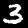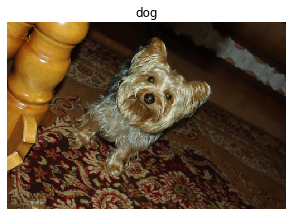Using Datasets, Pipeline, TfmdLists and Transform in computer vision

## Overview

In this tutorial, we look in depth at the middle level API for collecting data in computer vision. First we will see how to use:

• Transform to process the data
• Pipeline to composes transforms

Those are just functions with added functionality. For dataset processing, we will look in a second part at

• TfmdLists to apply one Pipeline of Tranforms on a collection of items
• Datasets to apply several Pipeline of Transforms on a collection of items in parallel and produce tuples

The general rule is to use TfmdLists when your transforms will output the tuple (input,target) and Datasets when you build separate Pipelines for each of your input(s)/target(s).

After this tutorial, you might be interested by the siamese tutorial that goes even more in depth in the data APIs, showing you how to write your custom types and how to customize the behavior of show_batch and show_results.

from fastai2.vision.all import *


## Processing data

Cleaning and processing data is one of the most time-consuming things in machine learning, which is why fastai tries to help you as much as it can. At its core, preparing the data for your model can be formalized as a sequence of transformations you apply to some raw items. For instance, in a classic image classification problem, we start with filenames. We have to open the corresponding images, resize them, convert them to tensors, maybe apply some kind of data augmentation, before we are ready to batch them. And that's just for the inputs of our model, for the targets, we need to extract the label of our filename and convert it to an integer.

This process needs to be somewhat reversible, because we often want to inspect our data to double check what we feed the model actually makes sense. That's why fastai represents all those operations by Transforms, which you can sometimes undo with a decode method.

### Transform

First we'll have a look at the basic steps using a single MNIST image. We'll start with a filename, and see step by step how it can be converted in to a labelled image that can be displayed and used for modeling. We use the usual untar_data to download our dataset (if necessary) and get all the image files:

source = untar_data(URLs.MNIST_TINY)/'train'
items = get_image_files(source)
fn = items; fn

Path('/home/sgugger/.fastai/data/mnist_tiny/train/3/7861.png')

We'll look at each Transform needed in turn. Here's how we can open an image file:

img = PILImage.create(fn); imgThen we can convert it to a C*H*W tensor (for channel x height x width, which is the convention in PyTorch):

tconv = ToTensor()
img = tconv(img)
img.shape,type(img)

(torch.Size([3, 28, 28]), fastai2.torch_core.TensorImage)

Now that's done, we can create our labels. First extracting the text label:

lbl = parent_label(fn); lbl

'3'

And then converting to an int for modeling:

tcat = Categorize(vocab=['3','7'])
lbl = tcat(lbl); lbl

TensorCategory(0)

We use decode to reverse transforms for display. Reversing the Categorize transform result in a class name we can display:

lbld = tcat.decode(lbl)
lbld

'3'

### Pipeline

We can compose our image steps using Pipeline:

pipe = Pipeline([PILImage.create,tconv])
img = pipe(fn)
img.shape

torch.Size([3, 28, 28])

A Pipeline can decode and show an item.

pipe.show(img, figsize=(1,1), cmap='Greys');The show method works behind the scenes with types. Transforms will make sure the type of an element they receive is preserved. Here PILImage.create returns a PILImage, which knows how to show itself. tconv converts it to a TensorImage, which also knows how to show itself.

type(img)

fastai2.torch_core.TensorImage

Those types are also used to enable different behaviors depending on the input received (for instance you don't do data augmentation the same way on an image, a segmentation mask or a bounding box).

### Creating your own Transform

Creating your own Transform is way easier than you think. In fact, each time you have passed a label function to the data block API or to ImageDataLoaders.from_name_func, you have created a Transform without knowing it. At its base, a Transform is just a function. Let's show how you can easily add a transform by implementing one that wraps a data augmentation from the albumentations library.

First things first, you will need to install the albumentations library. Uncomment the following cell to do so if needed:




Then it's going to be easier to see the result of the transform on a color image bigger than the mnist one we had before, so let's load something from the PETS dataset.

source = untar_data(URLs.PETS)
items = get_image_files(source/"images")


We can still open it with PILIlmage.create:

img = PILImage.create(items)
imgWe will show how to wrap one transform, but you can as easily wrap any set of transforms you wrapped in a Compose method. Here let's do some ShiftScaleRotate:

from albumentations import ShiftScaleRotate


The albumentations transform work on numpy images, so we just convert our PILImage to a numpy array before wrapping it back in PILImage.create (this function takes filenames as well as arrays or tensors).

aug = ShiftScaleRotate(p=1)
def aug_tfm(img):
np_img = np.array(img)
aug_img = aug(image=np_img)['image']
return PILImage.create(aug_img)

aug_tfm(img)We can pass this function each time a Transform is expected and the fastai library will automatically do the conversion. That's because you can directly pass such a function to create a Transform:

tfm = Transform(aug_tfm)


If you have some state in your transform, you might want to create a subclass of Transform. In that case, the function you want to apply should be written in the encodes method (the same way you implement forward for PyTorch module):

class AlbumentationsTransform(Transform):
def __init__(self, aug): self.aug = aug
def encodes(self, img: PILImage):
aug_img = self.aug(image=np.array(img))['image']
return PILImage.create(aug_img)


We also added a type annotation: this will make sure this transform is only applied to PILImages and their subclasses. For any other object, it won't do anything. You can also write as many encodes method you want with different type-annotations and the Transform will properly dispatch the objects it receives.

This is because in practice, the transform is often applied as an item_tfms (or a batch_tfms) that you pass in the data block API. Those items are a tuple of objects of different types, and the transform may have different behaviors on each part of the tuple.

Let's check here how this works:

tfm = AlbumentationsTransform(ShiftScaleRotate(p=1))
a,b = tfm((img, 'dog'))
show_image(a, title=b);The transform was applied over the tuple (img, "dog"). img is a PILImage, so it applied the encodes method we wrote. "dog" is a string, so the transform did nothing to it.

Sometimes however, you need your transform to take your tuple as whole: for instance albumentations is applied simultaneously on images and segmentation masks. In this case you need to subclass ItemTransfrom instead of Transform. Let's see how this works:

cv_source = untar_data(URLs.CAMVID_TINY)
cv_items = get_image_files(cv_source/'images')
img = PILImage.create(cv_items)
ax = img.show()We then write a subclass of ItemTransform that can wrap any albumentations augmentation transform, but only for a segmentation problem:

class SegmentationAlbumentationsTransform(ItemTransform):
def __init__(self, aug): self.aug = aug
def encodes(self, x):

And we can check how it gets applied on the tuple (img, mask). This means you can pass it as an item_tfms in any segmentation problem.
tfm = SegmentationAlbumentationsTransform(ShiftScaleRotate(p=1))